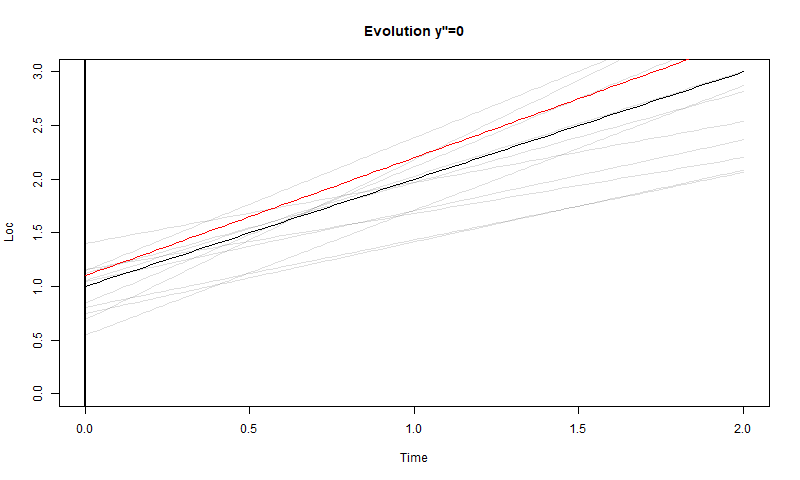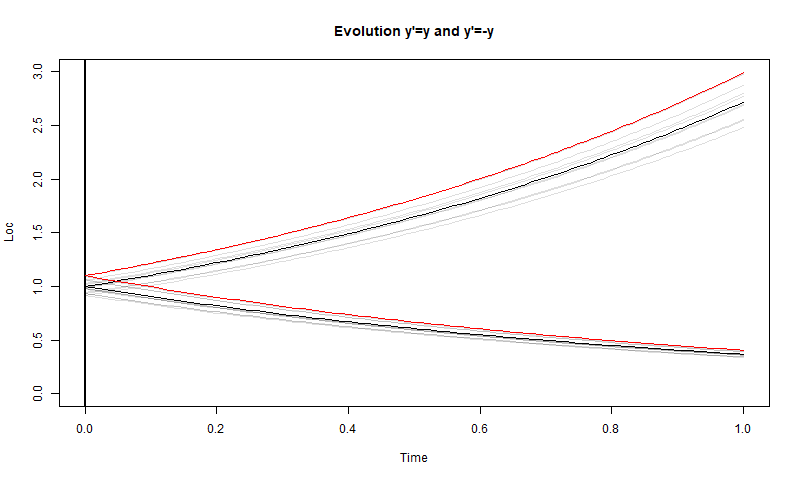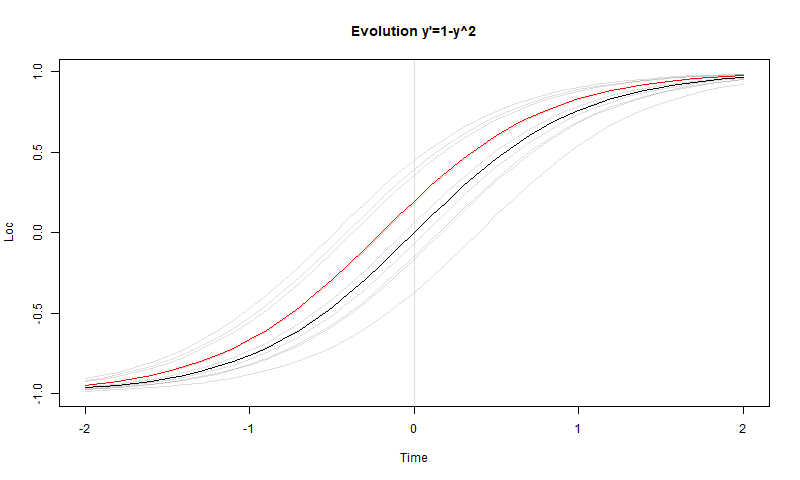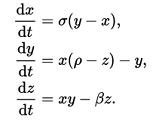# How error propagation works with differential equations (and GCMs)

guest post by Nick Stokes

There has been a lot of discussion lately of error propagation in climate models, eg here and here. I have spent much of my professional life in computational fluid dynamics, dealing with exactly that problem. GCM’s are a special kind of CFD, and both are applications of the numerical solution of differential equations (DEs). Propagation of error in DE’s is a central concern. It is usually described under the heading of instability, which is what happens when errors grow rapidly, usually due to a design fault in the program.

So first I should say what error means here. It is just a discrepancy between a number that arises in the calculation, and what you believe is the true number. It doesn’t matter for DE solution why you think it is wrong; all that matters is what the iterative calculation then does with the difference. That is the propagation of error.

A general linear equation in time can be formulated as

y’ = A(t)*y+f(t) ……….(1)
y(t) could be just one variable or a large vector (as in GCMs); A(t) will be a corresponding matrix, and f(t) could be some external driver, or a set of perturbations (error). The y’ means time derivative. With a non-linear system such as Navier-Stokes, A could be a function of y, but this dependence is small locally (in space and time) for a region; the basics of error propagation follow from the linearised version.

I’ll start with some bits of DE theory that you can skip (I’ll get more specific soon). If you have another solution z which is the solution following an error, then the difference satisfies

(y-z)’=A*(y-z)
The dependence on f(t) has gone. Error propagation is determined by the homogeneous part y’=A*y.

You can write down the solutions of this equation explicitly:

y(t) = W(t)*a, W(t) = exp(∫ A(u) du )
where the exp() is in general a matrix exponential, and the integral is from starting time 0 to t. Then a is a vector representing the initial state, where the error will appear, and the exponential determines how it is propagated.

You can get a long way by just analysing a single error, because the system is linear and instances can be added (superposed). But what if there is a string of sequential errors? That corresponds to the original inhomogeneous equation, where f(t) is some kind of random variable. So then we would like a solution of the inhomogeneous equation. This is

y(t) = W(t) ∫ W-1(u) f(u) du, where W(t)=exp(∫ A(v) dv ), and integrals are from 0 to t
To get the general solution, you can add any solution of the homogeneous equation.

For the particular case where A=0, W is the identity, and the solution is a random walk. But only in that particular case. Generally, it is something very different. I’ll describe some special cases, in one or few variable. In each case I show a plot with a solution in black, a perturbed solution in red, and a few random solutions in pale grey for context.

#### Special case 1: y’=0This is the simplest differential equation you can have. It says no change; everything stays constant. Every error you make continues in the solution, but doesn’t grow or shrink. It is of interest, though, in that if you keep making errors, the result is a random walk.

#### Special case 2: y”=0The case of no acceleration. Now if there is an error in the velocity, the error in location will keep growing. Already different, and already the simple random walk solution for successive errors doesn’t work. The steps of the walk would expand with time.

#### Special case 3: y’=c*y

where c is a constant. If c>0, the solutions are growing exponentials. The errors are also solutions, so they grow exponentially. This is a case very important to DE practice, because it is the mode of instability. For truly linear equations the errors increase in proportion to the solution, and so maybe don’t matter much. But for CFD it is usually a blow-up.But there are simplifications, too. For the case of continuous errors, the earlier ones have grown a lot by the time the later ones get started, and really are the only ones that count. So it loses the character of random walk, because of the skewed weighting.

If c<0, the situation is reversed (in fact, it corresponds to above with time reversed). Both the solutions and the errors diminish. For continuously created errors, this has a kind of reverse simplifying effect. Only the most recent errors count. But if they do not reduce in magnitude while the solutions do, then they will overwhelm the solutions, not because of growing, but just starting big. That is why you couldn’t calculate a diminishing solution in fixed point arithmetic, for example.

This special case is important, because it corresponds to the behaviour of eigenvalues in the general solution matrix W. A single positive eigenvalue of A can produce growing solutions which, started from any error, will grow and become dominant. Conversely the many solutions that correspond to negative eigenvalues will diminish and have no continuing effect.

#### Special case 4: Non-linear y’=1-y2

Just looking at linear equations gives an oversimplified view where errors and solutions change in proportion. The solutions of this equation are the functions tanh(t+a) and coth(t+a), for arbitrary a. They tend to 1 as t→∞ and to -1 as t→-∞. Convergence is exponential. So an error made near t=-1 will grow rapidly for a while, then plateau, then diminish, eventually rapidly and to zero.#### Special case 5: the Lorenz butterfly

This is the poster child for vigorous error propagation. It leads to chaos, which I’ll say more about. But there is a lot to be learnt from analysis. I have written about the Lorenz attractor here and in posts linked there. At that link you can see a gadget that will allow you to generate trajectories from arbitrary start points and finish times, and to see the results in 3D using webGL. A typical view is like thisLorenz derived his equations to represent a very simple climate model. They are:The parameters are conventionally σ=10, β=8/3, ρ=28. My view above is in the x-z plane and emphasises symmetry. There are three stationary points of the equations, 1 at (0,0,0),(a, a, 27)and,(-a, -a, 27) where a = sqrt(72). The last two are centres of the wings. Near the centres, the equations linearise to give a solution which is a logarithmic spiral. You can think of it as a version of y’=a*y, where a is complex with small positive real part. So trajectories spiral outward, and at this stage errors will propagate with exponential increase. I have shown the trajectories on the plot with rainbow colors, so you can see where the bands repeat, and how the colors gradually separate from each other. Paths near the wing but not on it are drawn rapidly toward the wing.

As the paths move away from the centres, the linear relation erodes, but really fails approaching z=0. Then the paths pass around that axis, also dipping towards z=0. This brings them into the region of attraction of the other wing, and they drop onto it. This is where much mixing occurs, because paths that were only moderately far apart fall onto very different bands of the log spiral of that wing. If one falls closer to the centre than the other, it will be several laps behind, and worse, velocities drop to zero toward the centre. Once on the other wing, paths gradually spiral outward toward z=0, and repeat.

Is the Pope Catholic? you might ask. But chaos is not bad, and we live with it all the time. There is a lot of structure to the Lorenz attractor, and if you saw a whole lot of random points and paths sorting themselves out into this shape, I think you would marvel not at the chaos but the order.

In fact we deal with information in the absence of solution paths all the time. A shop functions perfectly well even though it can’t trace which coins came from which customer. More scientifically, think of a cylinder of gas molecules. Computationally, it is impossible to follow their paths. But we know a lot about gas behaviour, and can design efficient ICE’s, for example, without tracking molecules. In fact, we can infer almost everything we want to know from statistical mechanics that started with Maxwell/Boltzmann.

CFD embodies chaos, and it is part of the way it works. People normally think of turbulence there, but it would be chaotic even without it. CFD solutions quickly lose detailed memory of initial conditions, but that is a positive, because in practical flow we never knew them anyway. Real flow has the same feature as its computational analogue, as one would wish. If it did depend on initial conditions that we could never know, that would be a problem.

So you might do wind tunnel tests to determine lift and drag of a wing design. You never know initial conditions in tunnel or in flight but it doesn’t matter. In CFD you’d start with initial conditions, but they soon get forgotten. Just as well.

#### GCMs and chaos

GCMs are CFD and also cannot track paths. The same loss of initial information occurs on another scale. GCMs, operating as weather forecasts, can track the scale of things we call weather for a few days, but not further, for essentially the same reasons. But, like CFD, they can generate longer term solutions that represent the response to the balance of mass, momentum and energy over the same longer term. These are the climate solutions. Just as we can have a gas law which gives bulk properties of molecules that move in ways we can’t predict, so GCMs give information about climate with weather we can’t predict.

#### What is done in practice? Ensembles!

Analysis of error in CFD and GCMs is normally done to design for stability. It gets too complicated for quantitative tracing of error, and so a more rigorous and comprehensive solution is used, which is … just do it. If you want to know how a system responds to error, make one and see. In CFD, where a major source of error is the spatial discretisation, a common technique is to search for grid invariance. That is, solve with finer grids until refinement makes no difference.

With weather forecasting, a standard method is use of ensembles. If you are unsure of input values, try a range and see what range of output you get. And this is done with GCMs. Of course there the runs are costlier, and so they can’t do a full range of variations with each run. On the other hand, GCM’s are generally surveying the same climate future with just different scenarios. So any moderate degree of ensemble use will accumulate the necessary information.

Another thing to remember about ensemble use in GCM’s is this. You don’t have to worry about testing a million different possible errors. The reason is related to the loss of initial information. Very quickly one error starts to look pretty much like another. This is the filtering that results from the vary large eigenspace of modes that are damped by viscosity and other diffusion. It is only the effect of error on a quite small space of possible solutions that matters.

If you look at the KNMI CMIP 5 table of GCM results, you’ll see a whole lot of models, scenarios and result types. But if you look at the small number beside each radio button, it is the ensemble range. Sometimes it is only one – you don’t have to do an ensemble in every case. But very often it is 5,6 or even 10, just for 1 program. CMIP has a special notation for recording whether the ensembles are varying just initial conditions or some parameter.

#### Conclusion

Error propagation is very important in differential equations, and is very much a property of the equation. You can’t analyse without taking that into account. Fast growing errors are the main cause of instability, and must be attended to. The best way to test error propagation, if computing resources are adequate, is by an ensemble method, where a range of perturbations are made. This is done with earth models, both forecasting and climate.

#### Appendix – emulating GCMs

One criticised feature of Pat Frank’s paper was the use of a simplified equation (1) which was subjected to error analysis in place of the more complex GCMs. The justification given was that it emulated GCM solutions (actually an average). Is this OK?

Given a solution f(t) of a GCM, you can actually emulate it perfectly with a huge variety of DEs. For any coefficient matrix A(t), the equation

y’ = A*y + f’ – A*f

has y=f as a solution. A perfect emulator. But as I showed above, the error propagation is given by the homogeneous part y’ = A*y. And that could be anything at all, depending on choice of A. Sharing a common solution does not mean that two equations share error propagation. So it’s not OK.

via Watts Up With That?

https://ift.tt/34NK8az

September 16, 2019 at 08:34PM